Spectral properties of endohedrally confined helium atom
Department of Physics, School of Physics and Technology, Wuhan University, Wuhan 430072, China

Corresponding author. E-mail: qhx@whu.edu.cn

*Project supported by the National Natural Science Foundation of China (Grant No. 11274246.)

Abstract

Based on the B-spline basis method, the properties of the helium atom confined inside an endohedral environment, such as buckminster fullerene, are studied. In our calculations, the endohedral environment is a parabolic potential well. In this situation, the phenomenon of “mirror collapse” is exhibited for energy levels of a confined helium atom. The “giant resonance” of oscillator strength of the dipole transition emerges with the variation of depth of the confining well. The physical mechanisms of these phenomena are analyzed in this paper.

PACS: 31.10.+z; 31.15.–p
Keyword: B-spline; fullerene; mirror collapse; giant resonance
1. Introduction

With the success of La@ C60 preparation, the experimental research has successively obtained K@ C60,  He@ C60,  and Ne@ C60,  and so on. In such an environment, which is a double-well of the atomic potential, some novel properties of atoms appear. In the theoretical research of endohedrally confined atoms,  only Connerade, [7, 9, 11, 13] Qiao,  and Zhang have focused on their spectral properties. Meanwhile, some researchers have done some experiments on the spectral properties of endohedrally confined atoms. In our work, a B-spline functions method is applied to calculate the energy level and the dipole oscillator strength of endohedrally confined helium, when the square potential well is changed into a parabolic potential one. The causes of the “ mirror collapse” and “ giant resonance” phenomena are analyzed.

This paper is organized as follows. In Section  2, the confined helium model and the B-spline functions are introduced. The results of energy levels and oscillator strength of the endohedrally confined helium are given in Section  3. This paper is closed by the conclusions in Section  4.

2. Method

For a helium atom in an endohedral environment, the Hamiltonian is: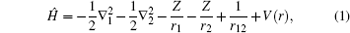where V(r) is the attractive potential shell. In this paper, the shape of the attractive potential is changed to soften the endohedral environment. The attractive potential shell is defined as a parabola form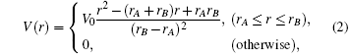where the parameters rA and rB are the inner and outer radii of the confining potential well, and the values are 5.75  a.u. (the unit a.u. is the abbreviation of atomic unit) and 7.64  a.u.,  respectively. In quantum mechanics, the equivalent potential of an electron is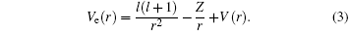Figure  1 shows the radial distribution of the equivalent potential of the 1P1 states. It is obviously a double well structure. The Coulomb well is called the inner well and the confining well is called the outer well. The parameter V0 is the depth of the outer well.

 Figure Option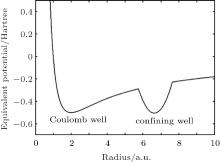Fig.  1. Equivalent potential curve of the 1P1 state. The unit 1  Hartree = 27.2114  eV.

Normally, the radial wave-functions are expanded in three spatial ranges: 0 < rrA, rA < rrB, and rB < r ≤ ∞ . In this paper, B-spline functions are used to expand the wave-functions and the boundary conditions are satisfied very simply.

With the order k, the B-spline functions are defined as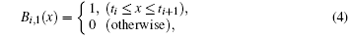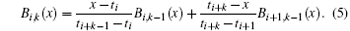Here, {ti} is the knots sequence set on the x axis. In our work, the order k is set to 7, so the knots sequence is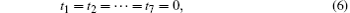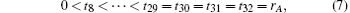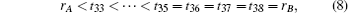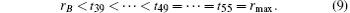The basis set is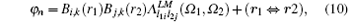which is used to expand the wave-functions of the system. From the Schrö dinger equations, the matrix equation is obtained by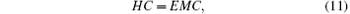where H and M are respectively the Hamiltonian and overlap matrix. E and C are respectively the eigenvalues and eigenvectors, which can be obtained by solving the matrix equation  (11).

In quantum mechanics, the oscillator strength of a dipole transition is calculated by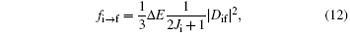where Δ E = | EfEi| , Ji is the initial state’ s total angular quantum number, Dif is the element of the dipole transition matrix and is defined by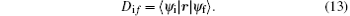As a consequence, for a specific dipole transition, the oscillator strength is determined by two terms: the energy difference and the square of the dipole transition matrix element.

3. Results and discussion

By solving Eqs.  (1) and (11), the energy levels of the endohedrally confined helium have been calculated. In our work, 11760 basis sets are included in the expansion of the wave function. As a validation of the B-spline functions methods, we get the ground state energy to − 2.903703  Hartree in the case that the depth of the outer potential well is 0 Hartree. The reliability of the method was verified.

3.1. Energy levels

In our calculations, V0 is taken from 0 to 6  Hartree in order to study the helium atom confined in buckminster fullerene more generally. The results of the calculation of the energy levels of the 1S0 and 1P1 states of a confined helium, as a function of the depth of the confining well, are shown in Fig.  2 and Fig.  3.

 Figure Option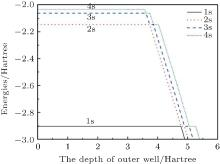Fig.  2. 1S0 states’ energy levels for the helium confined in an attractive well, as a function of the depth of the outer well, with rA = 5.75  a.u. and rB = 7.64  a.u.

 Figure Option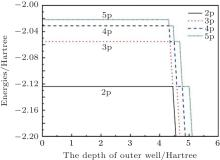Fig.  3. 1P1 states’ energy levels for the helium confined in an attractive well, as a function of the depth of the outer well, with rA = 5.75  a.u. and rB = 7.64  a.u.

As shown in Fig.  2 and Fig.  3, the energy levels of the 1S0 and 1P1 states of helium reveal similar phenomena. Now, as an example, the 1S0 states are used to describe the phenomena. It can be seen that a few of 1S0 states hold the energies of an unconfined helium atom when V0 is small. It seems that the outer confining well has no effect on them. The phenomenon can be understood by the distribution of the radial wave-functions of these 1S0 states. Figure  6(a) shows the one-body densities of the 11S0 and 21S0 states, which can represent the distributions of these states, with V0 = 1.0  Hartree. This reveals that the radial wave-functions of these 1S0 states are almost zero in the range of the outer well. So the outer confining well has no effect on them. New 1S0 states emerge when the depth of the outer confining well continues to increase, and their radial wave-functions are distributed in the range of the outer well. Thus, new states are bound in attractive confining wells, but the original states are still bound in Coulomb wells. The new 1S0 states will change the existing energy sorting. For example, when a new 11S0 state emerges, the original 11S0 state will become a 21S0 state, and 21S0 states will become 31S0 states, and so on.

In quantum mechanics, the condition that a system has bound states is that the depth of the confining well must achieve a certain value when the width of the confining well is fixed. In the double-well model, if the Coulomb potential is removed, the bound states will appear only when V0 ≥ 1.018  Hartree. To understand the influences of the Coulomb potential and the attractive confined well for the system energies, we use Table  1 and Table  2 to show the relationship between them. In Table  1, the energies of 1S0 states of free helium, only confining attractive potential helium (Coulomb potential is removed) and double-well helium, are given respectively, with V0 = 4.0  Hartree. The row of the 11S0 state shows that the energies of the 11S0 state of free helium and double-well helium are both − 2.9037  Hartree. It seems that the outer well has no effect on the 11S0 state. However, the 21S0 state of double-well helium is a new state, whose energy is − 2.3380  Hartree. This is the result of the cooperation of the Coulomb potential and the confining attractive potential.Table 1. The energies of 1S0 states of free helium, only confining potential helium (Coulomb potential is removed) and double-well helium, with V0 = 4.0  Hartree.Table 2. The VCoulomb and Vconfined are expectations of Coulomb potential and confining an attractive potential with V0 = 4.0  Hartree. R is the ratio of the expectation of confining attractive potential to the expectation of total potential.

In Table  2, the VCoulomb and Vonfined are expectations of Coulomb potential and confining attractive potential with V0 = 4.0  Hartree, respectively. R is the ratio of the expectation of the confining attractive potential to the expectation of the total potential. R is calculated by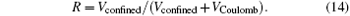It can be seen that the Coulomb potential makes the major contribution to the 11S0 state, and the confining attractive potential makes the dominant contribution to the 21S0 state. So the 11S0 state is the original state, and the confining attractive potential contribution can be ignored. The 21S0 state is a new state, and the Coulomb potential contribution is relatively small compared with the confining attractive potential. It is worth noting whether it is a new or an original state, the energy is the result of the combination of Coulomb potential and confining attractive potential.

Now let us focus on the analysis of the 1S0 states. The 11S0 state holds − 2.9037  Hartree, which is the 11S0 state energy of an unconfined helium atom. The first 1S0 state energy line is a horizontal line, as the depth of the outer attractive well increases until its depth reaches to − 4.773  Hartree. Then, the 11S0 state energy decreases very quickly, and its energy shows linear decreasing with the deepening of the outer confining well. To understand this phenomenon, the one-body density of the 11S0 state is calculated, as a function of the outer attractive well. For V0 = − 4.771  Hartree, the one-body density of the 11S0 state is distributed around the nucleus, which indicates the first 1S0 state is a free helium ground state, which can be seen from Fig.  6(b). For V0 = − 4.773  Hartree, the figure  6(c) shows that the one-body density of the 11S0 state is distributed around the nucleus and the confining well, indicating that the 11S0 state is the compound of a confining state and an unconfined helium ground state. In Fig.  6(d) with V0 = − 4.775  Hartree, the one-body density of the 11S0 state is distributed in the range of the outer confining well, indicating that the first 1S0 state is a bound state. The second 1S0 state has a similar behavior as the first 1S0 state has shown formerly. In Fig.  2, its energy holds − 2.1459  Hartree when V0 is small, which is the value of the 21S0 state energy of the unconfined helium, and the state stays in the Coulomb well. At the point V0 = − 3.728  Hartree, the energy of the second 1S0 state suddenly decreases and continues to decrease with the deepening of the outer confining well till V0 = − 4.773  Hartree. The state “ collapses” into the confining well. Then, when V0 > − 4.773  Hartree, the state “ collapses” into Coulomb potential and stays there. At the point V0 = − 4.891  Hartree, the state “ collapses” into the outer attractive well again and stays there finally. Some other 1S0 and 1P1 states’ behaviors have the same phenomena, which are shown in Fig.  2 and Fig.  3, respectively.

The phenomenon of “ collapse” can also be understood from the ratio of the expectation of the confining attractive potential to the expectation of the total potential. Figure  4 shows that the ratio R is very small for the 11S0 state when V0 is small, which implies that the Coulomb potential is dominant for the 11S0 state. At the point V0 = − 4.773  Hartree, the ratio R suddenly increases, which indicates that the 11S0 state “ collapses” into the confining well. Then, the ratio R is a large value when V0 ≥ − 4.773  Hartree, indicating that the outer attractive well is dominant for the 11S0 state. The 21S0 state’ s ratio reveals the same phenomenon. The ratio R is small when the 21S0 state is bound in the Coulomb well, and it is very large when the 21S0 state is bound in the outer well. At the “ collapse” point, the ratio R suddenly changes. The phenomenon also exists in other states of 1S0 and 1P1, which are shown in Fig.  4 and Fig.  5.

 Figure Option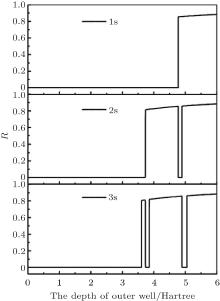Fig.  4. Ratio R of 1S0 states of the helium confined attractive well, changing the depth of the confining well. R is the ratio of the expectation of confined attractive potential to the expectation of total potential.

 Figure Option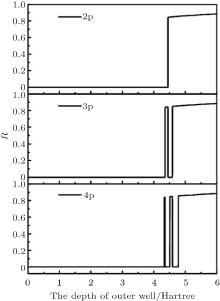Fig.  5. Ratio R of 1P1 states of the helium confined attractive well, changing the depth of the confining well. R is the ratio of the expectation of confined attractive potential to the expectation of total potential.

 Figure Option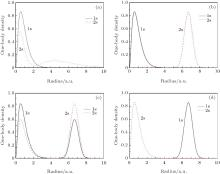Fig.  6. One-body densities of the 11S0 and 21S0 states of endohedrally confined helium atom. The depth of an attractive well is: (a) V0 = 1.0  Hartree, (b) V0 = 4.771  Hartree, (c) V0 = 4.773  Hartree, (d) V0 = 4.775  Hartree, respectively.

At the “ collapse” point, V0 = − 4.773  Hartree from Fig.  6(c), the first and the second 1S0 states are both strong mixtures of an unconfined helium state and a confined helium state, which leads to the result that their energy lines do not cross. Thus, at the avoided crossing point, V0 = − 4.773  Hartree, the first 1S0 state suddenly “ collapses” into the outer confining well, which previously is bound in the inner Coulomb well. Simultaneously, the second 1S0 state “ collapses” into the inner Coulomb well, which previously is bound in the outer confining well. The behaviors of the 1S0 states are called “ mirror collapse.” Figures  2 and 3 show that other states also have the same phenomena. The point of avoided crossing is a cut-off point of the horizontal lines and the oblique lines, which reveals that those states are respectively bound in the inner Coulomb well and the outer confining well. It is worth noting that all oblique lines are parallel, which demonstrates that the energies of all states bound in the outer attractive well decrease at the same rate, i.e. at the same rate as the deepening of the outer well.

3.2. Dipole oscillator strengths

The 1S01P1 dipole oscillator strengths of the endohedrally confined helium are calculated with Eqs.  (12) and (13) in our work. For a certain dipole transition, such as 11S0– 21P1 dipole transition, the energy difference is certain, so the dipole transition matrix element determines the oscillator strength. The dipole transition matrix element is related to the overlap of the wave-functions of the states involved in the transition. However, these wave functions are closely related to their one-body densities. So, the dipole transition matrix element is closely related to the overlap of one-body densities of the states involved in the transition.

The 11S0– 21P1 oscillator strengths are calculated and shown in Fig.  7(a) as functions of the depth of the confining potential well. When V0 is a small value, 11S0 and 21P1 states are both bound in Coulomb potential and the overlap of one-body density of them is normal, so that the oscillator strength has the same value corresponding to that of an unconfined helium atom, which can be seen from Fig.  7(a) and Fig.  7(b). At V0 = − 4.449  Hartree, the 21P1 state collapses into the outer well, but the 11S0 state is still bound in the Coulomb well. Then the oscillator strength suddenly decreases to a very small value from Fig.  7(a), but it is not zero. The reason is that the overlap of one-body densities of 11S0 and 21P1 states is close to zero from Fig.  7(c) with V0 = − 4.6  Hartree. By increasing the depth of the outer well, when the depth V0 = − 4.773  Hartree, the 11S0 state collapses into the outer well. In this case, from Fig.  7(d) with V0= − 4.9  Hartree, 11S0 and 21P1 states are all bound in the outer well so that their one-body densities overlap almost completely. So the value of oscillator strength is a large value, which is two orders of magnitude greater than the normal value of an unconfined helium atom. This phenomenon of very strong resonance enhancement of oscillator strength is called “ giant resonance.” At the same time, from Fig.  8(a), the 11S0 and 21P1 states are bound in the Coulomb well when V0 is small, so the oscillator strength is normal. At the point V0 = − 4.6  Hartree, the 11S0 state is still bound in the Coulomb well, but the 21P1 state is bound in the confining well. At that moment, the oscillator strength is very small. When V0 ≥ − 4.773  Hartree, the 11S0 and 21P1 states are both bound in the outer well, the oscillator strength is enhanced and the phenomenon of “ giant resonance” appears. Not only the oscillator strength of 11S0– 21P1 dipole transition, but also other dipole transitions have the phenomenon about “ giant resonance, ” for example, 11S0– 31P1 dipole transition, 21S0– 31P1 dipole transition, and so on. Figure  9 shows the results of the oscillator strength of 11S0– 31P1 dipole transition and the corresponding one-body densities of 11S0 and 31P1 states in different depths of the outer attractive well. Figure  10 shows the results of the oscillator strengths of the dipole transitions of 21S0– 21P1 and 21S0– 31P1. Figures  8(b), 8(c), and 8(d) show the ratio R of states that are included in the dipole transitions, and corresponding to the 11S0– 31P1 dipole transition, 21S0– 31P1 dipole transition, and 21S0– 31P1 dipole transition, respectively.

 Figure Option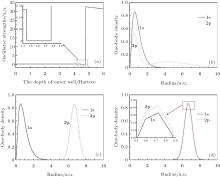Fig.  7. (a) The 11S0– 21P1 oscillator strengths of confined helium with changing depth of outer confining potential well; inset shows the enlarged figure of the dotted box. (b)– (d) one-body densities of 11S0 and 21P1 states of endohedrally confined helium atom. The depth of an attractive well is: (b) V0 = 4.4  Hartree, (c) V0 = 4.6  Hartree, (d) V0 = 4.9  Hartree, respectively.

 Figure Option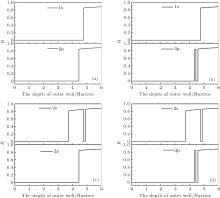Fig.  8. The ratio of the states involved in the dipole transition. (a) The dipole transition of 11S0– 21P1, (b) the dipole transition of 11S0– 31P1, (c) the dipole transition of 21S0– 21P1, (d) the dipole transition of 21S0– 31P1.

 Figure Option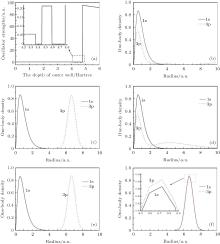Fig.  9. (a) The 11S0– 31P1 oscillator strengths of confined helium with changing depth of outer confining potential well; inset shows the enlarged figure of the dotted box. (b)– (e) one-body densities of 11S0 and 31P1 states of endohedrally confined helium atom. The depth of an attractive well is: (b) V0 = 4.3  Hartree, (c) V0 = 4.4  Hartree, (d) V0 = 4.5  Hartree, (e) V0 = 4.7  Hartree, (f) V0 = 4.9  Hartree, in which the inset shows the enlarged figure of the dotted box, respectively.

 Figure Option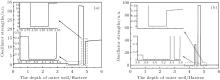Fig.  10. The 21S0 − 21P1 and 21S0– 31P1 oscillator strengths of confined helium with changing depth of the outer confining potential well; inset shows the enlarged figure of the dotted box. (a) The 21S0– 21P1 oscillator strength, (b) the 21S0– 31P1 oscillator strength.

4. Conclusion

The spectral properties of an endohedrally confined helium atom were calculated with the B-spline functions method. With the evolution of the energy spectrum as a function of the depth of the outer attractive well, the phenomenon of “ mirror collapse” exists in endohedrally confined helium. And the phenomenon of “ giant resonance” of the dipole oscillator strength appears with the increase of the depth of the confining well. These phenomena are due to the jumps of the wave-function between the Coulomb well and the confining attractive well, with the changing depth of the outer attractive well. It is readily apparent that the phenomena can also be understood from the major potential contribution changes between the Coulomb potential and the confining attractive potential, with the deepening of the outer attractive well.

The endohedral environment gives rise to some new questions about confined atoms. For example, the control of laser population, the prominent resonance in the photoionization cross section, and multiple photoionization, with endohedrally confined atoms. Related work is being pursued.

Reference
 1 Kroto H W, Allaf A W and Balm S P 1991 Chem. Rev. 91 1213 DOI:10.1021/cr00006a005 [Cited within:1] 2 Kroto H W, Mackay A L and Turner Get al. 1993 Phil. Trans. Roy. Soc. A 343 1 DOI:10.1098/rsta.1993.0035 [Cited within:1] 3 Whitehouse D B and Buckingham A D 1993 Chem. Phys. Lett. 207 332 DOI:10.1016/0009-2614(93)89009-7 [Cited within:1] 4 Cioslowski J and Nanayakkara A 1992 Phys. Rev. Lett. 69 2871 DOI:10.1103/PhysRevLett.69.2871 [Cited within:1] 5 Joslin C G, Gary C G and Goddard J Det al. 1993 Chem. Phys. Lett. 213 377 DOI:10.1016/0009-2614(93)85149-I [Cited within:1] 6 Jaskolski W 1996 Phys. Rep. 271 1 DOI:10.1016/0370-1573(95)00070-4 [Cited within:1] 7 Connerade J P 1997 J. Alloys Compd. 225 79 DOI:10.1016/S0925-8388(96)02820-4 [Cited within:1] 8 Varshni Y P 1997 J. Phys. B: At. Mol. Opt. Phys. 30 L589 DOI:10.1088/0953-4075/30/18/001 [Cited within:1] 9 Connerade J P and Dolmatov V K 1998 J. Phys. B: At. Mol. Opt. Phys. 31 3557 DOI:10.1088/0953-4075/31/16/009 [Cited within:1] 10 Decleva P, Alti G D and Fronzoni Get al. 1999 J. Phys. B: At. Mol. Opt. Phys. 32 4523 DOI:10.1088/0953-4075/32/18/311 [Cited within:1] 11 Connerade J P, Dolmatov V K and Manson S T 1999 J. Phys. B: At. Mol. Opt. Phys. 32 L395 DOI:10.1088/0953-4075/32/14/108 [Cited within:1] 12 Baltenkov A S 1999 J. Phys. B: At. Mol. Opt. Phys. 32 2745 DOI:10.1088/0953-4075/32/11/320 [Cited within:1] 13 Connerade J P 1999 J. Phys. B: At. Mol. Opt. Phys. 32 L239 DOI:10.1088/0953-4075/32/10/101 [Cited within:1] 14 Qiao H X and Li B W 2002 Commun. Theor. Phys. 37 475 DOI:10.1088/0253-6102/37/4/475 [Cited within:1] 15 Qiao H X, Shi T Y and Li B W 2002 Commun. Theor. Phys. 37 221 DOI:10.1088/0253-6102/37/2/221 [Cited within:1] 16 Qiao H X, Rao J G and Li B W 1997 Acta Phys. Sin. 46 2104(in Chinese) DOI:10.7498/aps.46.2104 [Cited within:1] 17 Zhang X Z, Jiao Z Y and Rao J G 2002 Nucl. Phys. Rev. 19 253(in Chinese) [Cited within:2] 18 Kolesnychenko O Y, ShKlyarevskii O I and Kempen H V 1999 Phys. Rev. Lett. 83 11 DOI:10.1103/PhysRevLett.83.2242 [Cited within:1] 19 Mitnik D M, Rand azzo J and Gasaneo G 2008 Phys. Rev. A 78 062501 DOI:10.1103/PhysRevA.78.062501 [Cited within:1] 20 Noh H, Yang J K, Vitebskiy I, Figotin A and Cao H 2010 Phys. Rev. A 82 013801 DOI:10.1103/PhysRevA.82.013801 [Cited within:1] 21 Luhr A, Al-Hujaj O A and Schmelcher P 2007 Phys. Rev. A 75 013403 DOI:10.1103/PhysRevA.75.013403 [Cited within:1] 22 Colavecchia F D, Gasaneo G and Mitnik D 2011 J. Phys. B: At. Mol. Opt. Phys. 44 817034 DOI:10.1155/2011/817034 [Cited within:1] 23 Iliew R, Etrich C, Pertsch T and Lederer F 2008 Phys. Rev. B 77 115124 DOI:10.1103/PhysRevB.77.115124 [Cited within:1] 24 Bachau H, Cormier E, Decleva P, Hansen J E and Martin F 2001 Rep. Prog. Phys. 64 1815 DOI:10.1088/0034-4885/64/12/205 [Cited within:1] 25 Xu Y B, Tan M Q and Becker U 1996 Phys. Rev. Lett. 76 3538 DOI:10.1103/PhysRevLett.76.3538 [Cited within:1] 26 Cabrera-Trujillo R and Cruz S A 2013 Phys. Rev. A 87 012502 DOI:10.1103/PhysRevA.87.012502 [Cited within:1] 27 Amusia M Y, BaltenKov A S and Chernysheva L V 2008 JETP Lett. 87 200 DOI:10.1134/S0021364008040048 [Cited within:1] 28 Kilcoyne A L D, Agilar A, Muller A, Schippers S, Cisneros C, Alna’Washi G, Aryal N B, Baral K K, Esteves D A, Thomas C M and Phaneuf R A 2010 Phys. Rev. Lett. 105 213001 DOI:10.1103/PhysRevLett.105.213001 [Cited within:1]